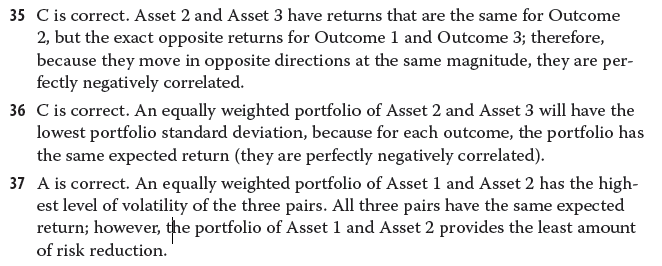# Portfolio Risk and Return (correlation between two assets)

Here is a case from the reading practice problem. I understand questions no 35 and 36. But I can’t figure out Q) 37

The AnswersCalculate the covariances for each pair.

you are right. I think I was just lazy and I needed someone to tell me
I calculated the
Cov.(1,2) = 0.012
Cov.(1,3) = -0.012
and it was already know that there is perfect -ve correlation between asset 2 and asset 3

so yes asset 1 and asset 2 provides the least risk reduction

Thank you S2000

My pleasure.

1 Like